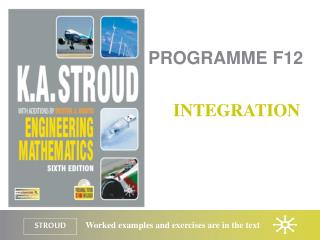Download PresentationPROGRAMME F12

# PROGRAMME F12

Download Presentation## PROGRAMME F12

- - - - - - - - - - - - - - - - - - - - - - - - - - - E N D - - - - - - - - - - - - - - - - - - - - - - - - - - -
##### Presentation Transcript

1. PROGRAMME F12 INTEGRATION

2. Programme F12: Integration Integration Standard integrals Integration of polynomial expressions Functions of a linear function of x Integration by partial fractions Areas under curves Integration as a summation

3. Programme F12: Integration Integration Standard integrals Integration of polynomial expressions Functions of a linear function of x Integration by partial fractions Areas under curves Integration as a summation

4. Programme F12: Integration Integration Constant of integration Integration is the reverse process of differentiation. For example: The integral of 4x3 is then written as: Its value is, however:

5. Programme F12: Integration Integration Standard integrals Integration of polynomial expressions Functions of a linear function of x Integration by partial fractions Areas under curves Integration as a summation

6. Programme F12: Integration Standard integrals Just as with derivatives we can construct a table of standard integrals:

7. Programme F12: Integration Integration Standard integrals Integration of polynomial expressions Functions of a linear function of x Integration by partial fractions Areas under curves Integration as a summation

8. Programme F12: Integration Integration of polynomial expressions Just as polynomials are differentiated term by term so they are integrated, also term by term. For example:

9. Programme F12: Integration Integration Standard integrals Integration of polynomial expressions Functions of a linear function of x Integration by partial fractions Areas under curves Integration as a summation

10. Programme F12: Integration Functions of a linear function of x To integrate we change the variable by letting u = ax + b so that du = a.dx. Substituting into the integral yields:

11. Programme F12: Integration Integration Standard integrals Integration of polynomial expressions Functions of a linear function of x Integration by partial fractions Areas under curves Integration as a summation

12. Programme F12: Integration Integration by partial fractions To integrate we note that so that:

13. Programme F12: Integration Integration Standard integrals Integration of polynomial expressions Functions of a linear function of x Integration by partial fractions Areas under curves Integration as a summation

14. Programme F12: Integration Areas under curves Area A, bounded by the curve y = f(x), the x-axis and the ordinates x = a and x = b, is given by: where

15. Programme F12: Integration Integration Standard integrals Integration of polynomial expressions Functions of a linear function of x Integration by partial fractions Areas under curves Integration as a summation

16. Programme F12: Integration Integration as a summation Dividing the area beneath a curve into rectangular strips of width x gives an approximation to the area beneath the curve which coincides with the area beneath the curve in the limit as the width of the strips goes to zero.

17. Programme F12: Integration Integration as a summation If the area is beneath the x-axis then the integral is negative.

18. Programme F12: Integration Integration as a summation The area between a curve an intersecting line The area enclosed between y1 = 25 – x2 and y2 = x + 13 is given as:

19. Programme F12: Integration Learning outcomes • Appreciate that integration is the reverse process of differentiation • Recognize the need for a constant of integration • Evaluate indefinite integrals of standard forms • Evaluate indefinite integrals of polynomials • Evaluate indefinite integrals of ‘functions of a linear function of x’ • Integrate by partial fractions • Appreciate the definite integral is a measure of an area under a curve • Evaluate definite integrals of standard forms • Use the definite integral to find areas between a curve and the horizontal axis • Use the definite integral to find areas between a curve and a given straight line